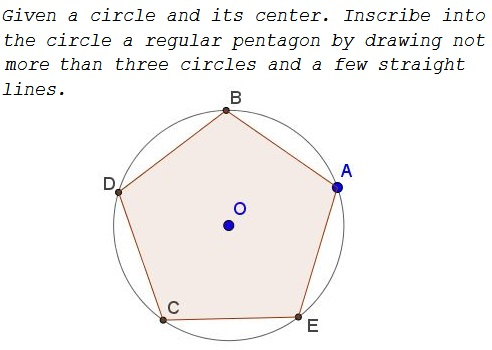# Regular Pentagon Construction by K. Knop

### Problem### Construction

1. Draw line $OA.\,$ Mark point $R,\,$ the second intersection of the line with the givem circle $O(A).$

2. Draw the circle $R(O)\,$ through $O\,$ centered at $R.\,$ Mark point $N,\,$ one of the intersections of the two circles.

3. Draw the circle $S(O),\,$ through $O\,$ centered at $S.\,$ Mark point $M\,$ - one of the intersections of $S(O)\,$ and $O(A).\,$ Choose the one nearest to $N.$

4. Draw line $MN\,$ and mark point $Q\,$ of its intersection with $OA.$

5. Draw circle $R(Q)\,$ and mark its intersections, say, $B\,$ and $E,\,$ with $O(A).$

6. Draw $BQ\,$ and $EQ\,$ and mark their intersections - $D\,$ and $C\,$ - with $O(A).\,$ $ABDCE\,$ is a regular pentagon.

### Proof of the construction

Assum circle $O(A)\,$ is defined by $x^2+y^2=1.\,$ Then $R(O)\,$ is defined by $(x+1)^2+y^2=1,\,$ and $S(O)\,$ by $(x+2)^2+y^2=4.$

We can find $\displaystyle M=\left(-\frac{1}{4},-\frac{\sqrt{15}}{4}\right)\,$ and $\displaystyle N=\left(-\frac{1}{2},-\frac{\sqrt{3}}{2}\right).\,$

With these $\displaystyle Q=\left(-\frac{3+\sqrt{5}}{2},0\right)\,$ such that $|QR|=\displaystyle \frac{1+\sqrt{5}}{2}=\varphi,\,$ the Golden ratio.

The rest of the proof is left as an exercise to the reader.

### Acknowledgment

The above problem comes from an uncommon site euclidea, devoted to the problems of Euclidean construction. The site and the problem have been brought to my attention by Konstantin Knop who also generously shared his construction.### Various Geometric Constructions

• How to Construct Tangents from a Point to a Circle
• How to Construct a Radical Axis
• Constructions Related To An Inaccessible Point
• Inscribing a regular pentagon in a circle - and proving it
• The Many Ways to Construct a Triangle and additional triangle facts
• Easy Construction of Bicentric Quadrilateral
• Easy Construction of Bicentric Quadrilateral II
• Star Construction of Shapes of Constant Width
• Four Construction Problems
• Geometric Construction with the Compass Alone
• Construction of n-gon from the midpoints of its sides
• Short Construction of the Geometric Mean
• Construction of a Polygon from Rotations and their Centers
• Squares Inscribed In a Triangle I
• Construction of a Cyclic Quadrilateral
• Circle of Apollonius
• Six Circles with Concurrent Pairwise Radical Axes
• Trisect Segment: 2 Circles, 4 Lines
• Tangent to Circle in Three Steps
•